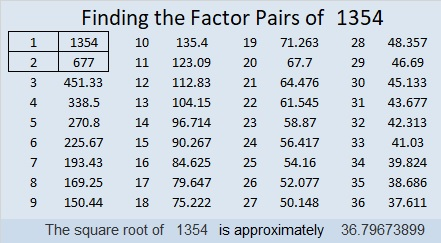# 1354 Solving a Level 5 Puzzle

What are the common factors of 16 and 4? Don’t guess which one to use. Use logic to figure it out as you find all the factors for this puzzle!Print the puzzles or type the solution in this excel file: 10 Factors 1347-1356

If you get stuck, you can watch this video:

Now I’ll share some information about the puzzle number, 1354:

• 1354 is a composite number.
• Prime factorization: 1354 = 2 × 677
• 1354 has no exponents greater than 1 in its prime factorization, so √1354 cannot be simplified.
• The exponents in the prime factorization are 1, and 1. Adding one to each exponent and multiplying we get (1 + 1)(1 + 1) = 2 × 2 = 4. Therefore 1354 has exactly 4 factors.
• The factors of 1354 are outlined with their factor pair partners in the graphic below.1354 is the sum of two squares:
27² + 25² = 1354

1354 is the hypotenuse of a Pythagorean triple:
104-1350-1354 which is 2 times (52-675-677)
and can also be calculated from 27² – 25², 2(27)(25), 27² + 25²

This site uses Akismet to reduce spam. Learn how your comment data is processed.